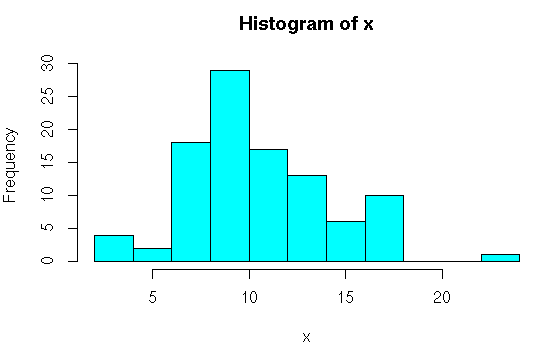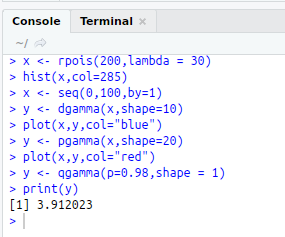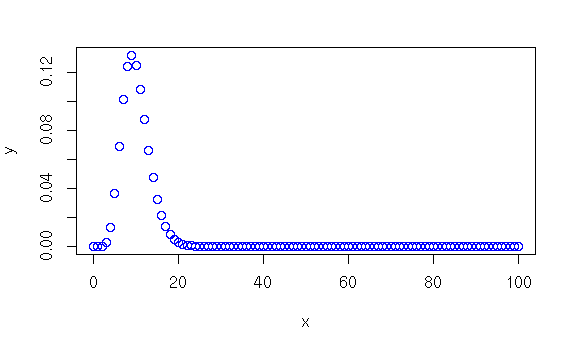• #5, First Floor, 4th Street Dr. Subbarayan Nagar Kodambakkam, Chennai-600 024 Landmark : Samiyar Madam
• pro@slogix.in
• +91- 81240 01111

### How to implement Gamma Distribution in R?

###### Description

To implement the gamma distribution using R programming.

#### Four main functions in binomial distribution:

dgamma(x, shape= )

Gives height of the probability distribution at each point pnbinom(x, shape= )

Gives the cumulative probability of an event qnbinom(p, shape= )

It takes the probability value and gives a number whose cumulative value matches the probability value.

rnbinom(x, shape= ) Used to generate random numbers whose distribution is binomial.

x -- vector

###### Sapmle Code

#Gamma Distribution
#dgamma
x<-seq(0,100,by=1)
y<-dgamma(x,shape=10)
plot(x,y,col=”blue”)

#pgamma
y<-pgamma(x,shape=20)
plot(x,y,col=”red”)

#qgamma
y<-qgamma(p=0.98,shape = 1)
print(y)

#rgamma
x<-rgamma(100,shape=10)
hist(x,col=285)

###### Screenshots